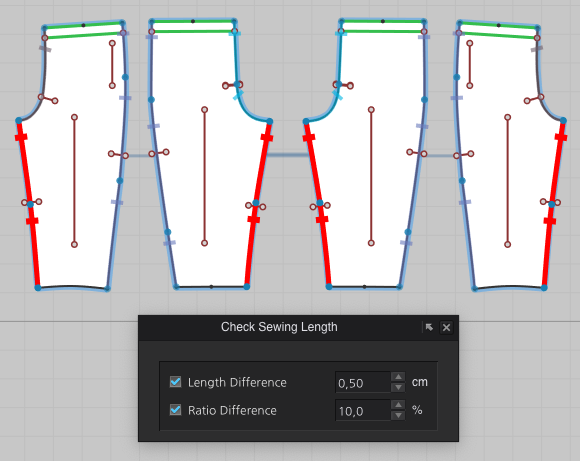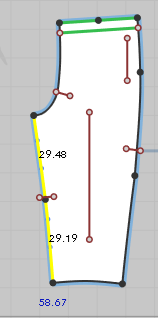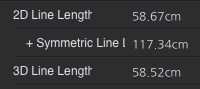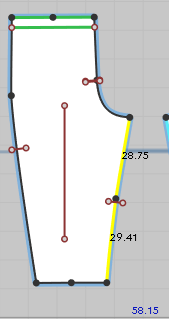CLO Help Center

## Check Sewing Length

I have a seam where the 3D line lengths are exactly the same but the sewing line is red. It seems as if the sewing line measurement works based on the 2D line length? That doesn't make sense though as the stitching follows the curved line (3D) and not a straight segment (2D).

This is what I see:

1) sewing length shows red2) Select front inseam (in yellow), total length shows 58,67cm (in blue)3) 2D line length msmt corresponds and is 58.67cm. 3D line is 58.52cm.Then I check the back inseam.

4) (in yellow), total length shows 58,15cm (in blue).5) 2D line length msmt corresponds and is 58.15cm. 3D line is 58.52cm.I can see that the segments measurement in blue is the 2D line measurement. In this case the difference front/back is 0.52cm

The difference of 3D line measurement is 0cm. This being the sewing line I do not expect seams highlighted in red when checking sewing length.

So my conclusion is as mentioned above that sewing length is calculated based on 2D line length. As in this case it is 0.52cm so more than the acceptable 0.5cm.

Correct me if I am wrong?

Thanks a lot. Ana.